Science, Maths & Technology

### Become an OU studentEveryday maths 1 (Wales)

Start this free course now. Just create an account and sign in. Enrol and complete the course for a free statement of participation or digital badge if available.

# 4 Area

Area is the amount of space a flat shape takes up. You need to be able to calculate area if you ever need to order a carpet for your house, buy tiles for a kitchen or bathroom, or calculate how much paint to buy when redecorating.

This patio has paving slabs that are 1 metre square (each side is 1 metre). How many paving slabs are there on the patio?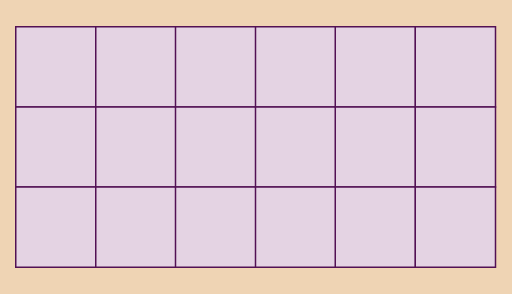Figure 20 Paving slabs

Area is measured in ‘square’ units. This means that the area is shown as the number of squares that would cover the surface. So if a patio covered with 18 squares that are 1 metre by 1 metre, the area is 18 square metres.

(If you count them, you will find there are 18 squares.)

Smaller areas would be measured in square centimetres. Larger areas can be measured in square kilometres or square miles.

You can work out the area of a rectangle, like the patio above, by multiplying the long side by the short side:

width × length = area

The patio is:

6 × 3 = 18 square metres

‘Square metres’ can also be written ‘sq m’ or ‘m2’.

## Example: The area of a table

Fran sees a rectangular dining table she likes. It measures 2 m by 1.5 m. What is the area of the table?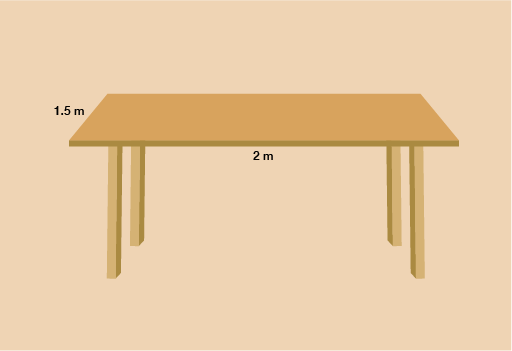Figure 21 A dining table

### Method

To find the area we need to multiply the length of the table by the width:

2 m × 1.5 m = 3 square metres

So the table is 3 m2.

## Activity 9: Area of a rectangle

Complete the following table by calculating the missing areas without using a calculator. You will have looked at methods for multiplying whole numbers and decimals [Tip: hold Ctrl and click a link to open it in a new tab. (Hide tip)] in Session 1. Show your answers in correct square units.

LengthWidthArea in square units (centimetres, metres, kilometres)
80 cm30 cm
To use this interactive functionality a free OU account is required. Sign in or register.
7 m4 m
To use this interactive functionality a free OU account is required. Sign in or register.
2.5 km2 km
To use this interactive functionality a free OU account is required. Sign in or register.
5.5 m2.4 m
To use this interactive functionality a free OU account is required. Sign in or register.
Interactive feature not available in single page view (see it in standard view).

LengthWidthArea in square units (centimetres, metres, kilometres)
80 cm30 cm2 400 cm Footnotes   2
7 m4 m28 m Footnotes   2
2.5 km2 km5 km Footnotes   2
5.5 m2.4 m13.2 m Footnotes   2

Hint: Always use the same units for both sides. Sometimes the length and width of the rectangle will be given in different units. They must be in the same unit before you can calculate area, so you may need to convert one side to the same units as the other side.

## Example: The area of a rug

How much backing fabric is needed for this rug?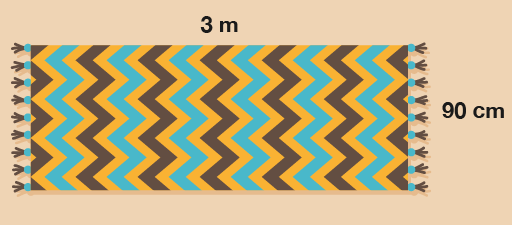Figure 22 A rug

### Method

To find the answer, you need to work out the width multiplied by the length.

• 90 cm × 3 m = area

First, you need to convert the width to metres so that both sides are in the same units. 90 cm is the same as 0.9 m, so the calculation is:

• 0.9 × 3 = area = 2.7 m2

Now try the following activity. You need to carry out the calculations without a calculator, but you can double-check your answers on a calculator if needed. If you need a reminder about how to multiply whole numbers or decimal numbers without a calculator, please look back at Session 1 first.

Remember to check if you need to convert the measurements before calculating the area.

## Activity 10: Finding the area

1. How much plastic sheeting do you need to cover this pond for the winter?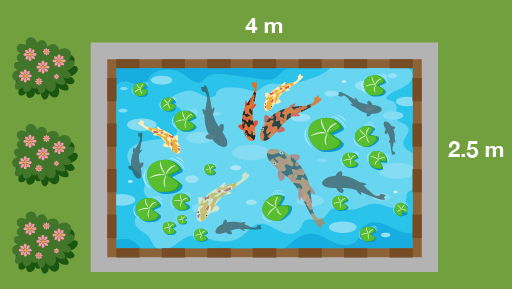Figure 23 A pond
2. One bag of gravel will cover half a square metre of ground. How many bags do you need to cover this driveway?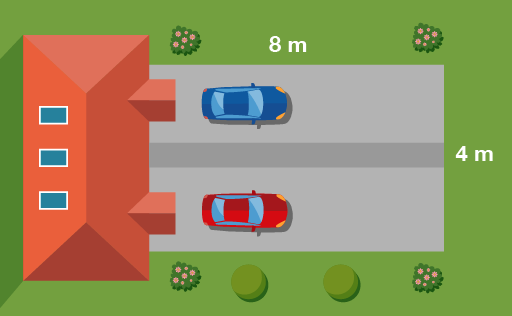Figure 24 A driveway
3. A biologist is studying yeast growth. In the sample area shown below in purple the biologist found 80 yeast. What would go in the missing spaces in her recording sheet, as marked with a question mark?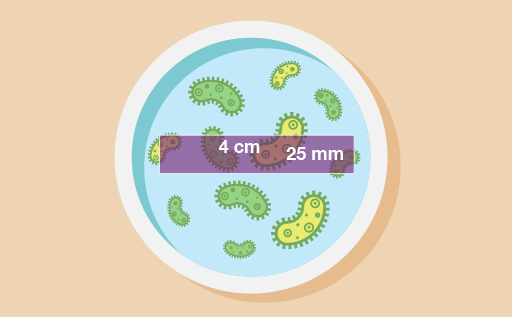Figure 25 A petri dish
 Sample area no. 21 Date 17 October Yeast count 80 Sample dimensions ? cm × ? cm Sample area ? cm Footnotes   2 Yeast/cm Footnotes   2 ?
1. How large is this area of forestry land?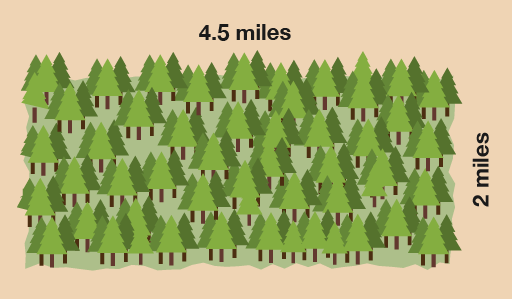Figure 26 A forest

1. The plastic sheeting needs to be:

• 2.5 × 4 = 10 m2
2. First you need to work out the area of the driveway:

• 8 × 4 = 32 m2

If each bag covers half a square metre, you will need two bags for each square metre:

• 32 × 2 = 64 bags
3. First you need to change the width to centimetres. 25 mm is the same as 2.5 cm. Then you can work out the area:

• 2.5 × 4 = 10 cm2

There are 80 yeast, so the amount of yeast per square centimetre (yeast/cm2) is:

• 80 ÷ 10 = 8 yeast per cm2

The recording sheet should look like this:

 Sample area no. 21 Date 17 October Yeast count 80 Sample dimensions 2.5 cm × 4 cm Sample area 10 cm Footnotes   2 Yeast/cm Footnotes   2 8
1. The area of forestry land is:

• 4.5 × 2 = 9 square miles

## Summary

In this section you have learned how to work out the area of a rectangular shape.